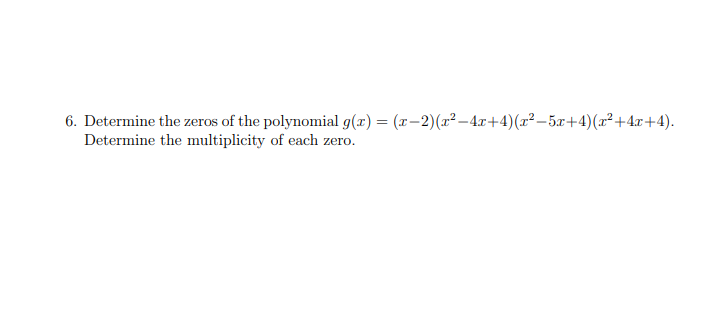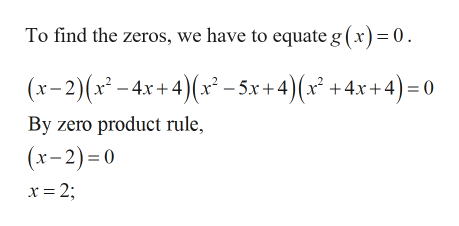# 6. Determine the zeros of the polynomial g(x) = (x-2)(z2-4r+4) (x2-5r+4)(x2+4x+4)Determine the multiplicity of each zero

Question
4 views

question 6help_outlineImage Transcriptionclose6. Determine the zeros of the polynomial g(x) = (x-2)(z2-4r+4) (x2-5r+4)(x2+4x+4) Determine the multiplicity of each zero fullscreen
check_circle

Step 1

Given:

Step 2

Calculation:help_outlineImage TranscriptioncloseTo find the zeros, we have to equate g (x)= 0 x-2)(x-4x+4)(x-5x+4)(x +4x+4)=0 By zero product rule, (x-2) 0 x 2; fullscreen
Step 3

### Want to see the full answer?

See Solution

#### Want to see this answer and more?

Solutions are written by subject experts who are available 24/7. Questions are typically answered within 1 hour.*

See Solution
*Response times may vary by subject and question.
Tagged in

### Other